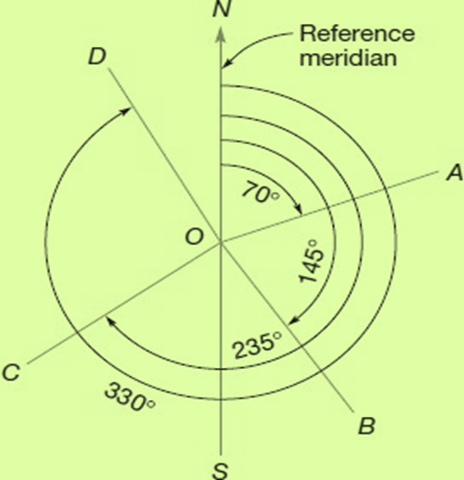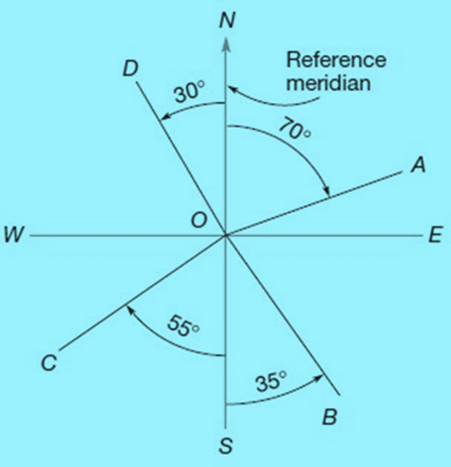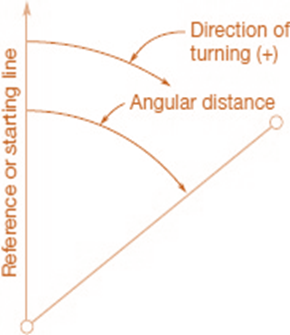WHAT IS AZIMUTH AND BEARINGS IN SURVEYING | CALCULATIONS - LCETED -lceted LCETED INSTITUTE FOR CIVIL ENGINEERS

## Jun 8, 2021

Introduction

The locations of the points and the orientation of the lines are usually determined by the observation of angles and directions. In the survey, the azimuth and bearings give directions. The angles measured in the survey are determined into horizontal or vertical, depending on the level at which it can be observed.

What is Azimuths In Surveying?

Azimuths are the horizontal angles found in the clockwise direction from any meridian reference. In aircraft surveillance, the azimuth is generally found from the north, but astronomers and the military use it as a reference directly to the south. Examples of azimuths observed from the north are shown in the figure below. As explained, they range in value from 0 to 360⁰. Thus OA's Azimuth 70⁰; of OB, 145⁰; OC, 235⁰; And OD, 330⁰. To avoid confusion it is necessary to mention in the field notes, at the beginning of the work, where the azimuths were found, whether from the north or south.Azimuth

Reverse direction by its back azimuth. In aerial surveying, forward azimuths are converted to back azimuths and vice versa by adding or subtracting 180⁰.

For example,

If the azimuth of OA is 60⁰

The azimuth of OA is 60⁰+180⁰ = 240⁰.

If the azimuth of OC is 225⁰

The azimuth of CO is 225⁰ - 180⁰ = 45⁰.

Azimuths can be read directly on a total station instrument's graduated circle after the instrument has been oriented properly.  Azimuths are used advantageously in boundary, topographic, control, and other kinds of surveys and computations.

What Is Bearings In Surveying?

Bearing is another system for indicating the directions of lines. The bearing reference of a line is defined as the acute horizontal angle between the meridian and the line.

For angles smaller than 90⁰ the angle is observed from north or south to east or west.

The letter N or S before the angle, and E or W following it, show the correct quadrant.

Therefore, a properly expressed bearing‌ has square letters and an angular value.Bearings

An example is the N80⁰E. In the figure below, all bearings in the quadrant NOE is measured clockwise from the meridian.

Thus the bearing N70⁰E of the OA line. All bearings in the Quadrant SOE are in the counterclockwise direction from the meridian, hence the OB S35⁰E.

Similarly, the bearing of OC is S55⁰W and OD, N30⁰W. When the lines are in cardinal directions, the bearing should be listed as "due north", "Dew East", "Dew South," or "Dew West"

COMPARISON OF AZIMUTHS AND BEARINGS

 AZIMUTHS BEARINGS Value differs from 0⁰ to 360⁰ Value differs from 0⁰ to 90⁰ Require only a numerical value Require numerical value and two letters It may be the geodetic, astronomic, magnetic, grid, assumed, forward or back. Same as azimuths Are measured clockwise only Are measured clockwise and counterclockwise Are measured either from north only or from south only on a particular survey Are measured from north and south QUADRANT FORMULAS FOR COMPUTING BEARING ANGLES FROM AZIMUTHS I (NE) Bearing = Azimuth II (SE) Bearing = 180⁰ - Azimuth III (SW) Bearing = Azimuth - 180⁰ IV (NW) Bearing = 360⁰ - Azimuth Example Directions For Lines In The Four Quadrants (Azimuths From North) 64⁰ N64⁰E 122⁰ S78⁰E 241⁰ S61⁰W 335⁰ N25⁰W

EXAMPLE:

The azimuth of a boundary line is 116⁰11’34”. Convert this to a bearing.

Solution:

The azimuth places the line in the southeast quadrant. Thus, the bearing angle is

180⁰ - 116⁰ 11’ 34” = 63° 48' 26''

And the equivalent bearing is S63° 48' 26''E

The first course of a boundary survey is written as N24⁰23’W. What is its equivalent azimuth?

Solution:

Since the bearing is in the northwest quadrant, the azimuth is

360⁰ - 24⁰ 23’ = 335°  37'  0''

COMPUTING AZIMUTHS

Most types of surveys, but especially those that employ traversing, require the computation of azimuths (or bearings).

Following Are The Basic Requirements To Determine An Angle

a.   Starting Line or Reference Point

b.   Turning Direction

c.    Angular DistanceBasic Requirements in determining an angle

Measurement Unit

The value of an angle is defined by the arbitrary. In the united states, the sexagesimal system used  and many other countries are based on degrees, minutes, and seconds, with the last unit, further divided decimally

In Europe, grad or gon is commonly used. Radians may be more appropriate in computer calculations, but the method of sexagesimal is consistently used in most surveys.

Kinds of Horizontal Angles

Most commonly observed in surveying are:

a.   Interior Angles

b.   Angles to the right

c.    Deflection Angles

FAQ

How do you convert azimuth to bearings?

Converting azimuths to quadrant bearings or vice versa is easy. For example, The azimuth of a boundary line is 116⁰11’34”. Convert this to a bearing. The azimuth places the line in the southeast quadrant. Thus, the bearing angle is 180⁰ - 116⁰ 11’ 34” = 63° 48' 26'' And the equivalent bearing is S63° 48' 26''E

What is the difference between azimuth and compass bearing?

Azimuth is the direction of travel expressed in degrees (135 degrees) as indicated by the compass. A bearing describes an angle or difference from a point. In compass, you use north and south for reference.

what direction Azimuth can measure?

Azimuth Are measured either from north only or from south only on a particular survey

If you find